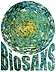Biological Small Angle ScatteringATSAS online  |  Forum  |  User information  |  EMBL Hamburg

datgnom4

# Manual

The following sections shortly describe how to run DATGNOM from the command-line on any of the supported platforms, the required input and the produced output files.

If you use results from DATGNOM in your own publication, please cite:

M.V. Petoukhov, P.V. Konarev, A.G. Kikhney and D.I. Svergun (2007) ATSAS 2.1 - towards automated and web-supported small-angle scattering data analysis. J. Appl. Cryst. 40, s223-s228

## Introduction

DATGNOM (formerly known as AUTOGNOM) is a tool for automated estimation of the maximum intramolecular distance Dmax, computation of the distance distribution function p(r) and of the smooth regularized scattering curve (the Fourier transform of p(r)). DATGNOM automatically obtains the p(r) function from an indirect Fourier transform of the scattering profile repeatedly using a regularization procedure implemented in the program GNOM.

The optimum Dmax is determined from automated comparison of the p(r) functions calculated at different Dmax values ranging from 2Rg to 4Rg (with a step of 0.1Rg), where Rg is the expected radius of gyration. The calculated p(r) functions and corresponding fits to the experimental curves are compared using the perceptual criteria of GNOM, where the smoothness of p(r), absence of systematic deviations in the fit, stability of the solution etc. are merged into a total quality estimate. The behaviour of p(r) near Dmax is taken into account to ensure that the p(r) function goes smoothly to zero.

## Running datgnom

Usage:

`\$ datgnom4 <DATAFILE> [OPTIONS]`

OPTIONS known by DATGNOM are described in next section, the required argument 'DATAFILE' in the section on input files.

### Command-line arguments and options

DATGNOM requires one command line argument DATAFILE which is a data filename, possibly with relative or absolute path components.

DATGNOM recognizes following command-line options:

Short optionLong optionDescription
-r --Rg Expected radius of gyration; typically from the Guinier approximation.
-s --skip How many first data points to skip, normally the first Guinier point minus one.
-o --output FILE Relative or absolute path to save the result; if not specified, the output filename will be the same as <DATAFILE> but with ".out" extension.
-v --version Print version information and exit.
-h --help Print a summary of arguments and options and exit.

### Runtime output

Dmax and Rg computed from the p(r) are printed to stdout.

## datgnom input file

DATAFILE must contain three columns: scattering vector, experimental intensity, experimental errors.

## datgnom output file

Same as GNOM output.

# Example

```\$ autorg bsa_003.dat -f table
File              Rg  stdev  I(0)    stdev  Guinier points   Quality
bsa_003.dat       3.03   1%  58.8       0%   39-135 ( 97)    85%

\$ datgnom bsa_003.dat --Rg 3.03 --skip 38
Dmax =    10.377749999999999       Total =   0.75569896756708321
Guinier =    3.0300000000000000       Gnom =    3.1076350783882152```

Here Dmax is the maximum intramolecular distance, Total is the total quality estimate, Guinier is the same value as the --Rg option, Gnom is the radius of gyration computed from the p(r). The output file will be named "bsa_003.out" because the --output option was not specified.